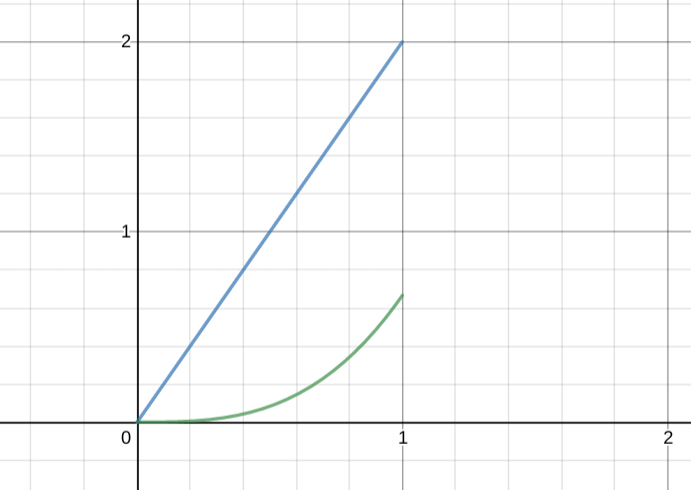# Introducing Stable Distributions

The story so far:

• There are lots of ways to put two functions together and get a new one out of the process.
• Addition is one of these ways. Multiplication is another.
• If you add two Gaussian functions together, you don’t get another Gaussian function.
• If you multiply two Gaussian functions together, guess what? You get another Gaussian function.
• Convolution is another way of combining two functions.
• If you convolve two Gaussian functions together, guess what? You get another Gaussian function.

In the last post I tried to explain why convolving two probability distributions produces the distribution of the sum of those variables. And that sum is guaranteed to also be a bell curve, as long as the distributions its made of come are distributed normally as well.

It’s worth stewing for a moment on what that means, because a lot of things that we care about can be thought of as sums of random variables.

One example is height. Take, for example, the height of a forest. I know nothing about the biology of forest height, but I did find a few figures online. (Yes, random searching.)

Here they are.Some of these plots look normal. Then again, some of them don’t. I don’t really care! Let’s pretend that forest height truly is normally distributed. Why would that be?

The thing is that there are a lot of factors that go into how tall a forest is height. There is underlying genetic variation in the families of trees that make a forest. There is underlying randomness is the environmental conditions where a forest grows, and “environmental conditions” is itself not a single factor but another collection of random variables — rain, soil, etc.

If we were going to make a list of factors that go into forest height how long do you think it would be before we had an exhaustive list? Hundreds of factors? Thousands?

Given all this, “forest height” really isn’t a single random variable. It’s a collection of random variables, not the way we think of a single coin flip.

(AND IS A COIN FLIP EVEN REALLY A SINGLE RANDOM EVENT??? OOOOOOOH DID I BLOW YOUR MIND?)

This all feels very complicated. How is it that a forest’s height has such a lovely normal distribution?

Given the math in the previous post we have a very tidy answer: forest height is thought of as a sum of random variables. Even if there are hundreds or thousands of underlying random variables that sum up to forest height, as long as all (most?) of them are themselves Gaussian, so will their sum, represented by the convolution of all those thousands of distributions.

(Note: we did not prove that convolution preserves the Gaussian nature of a distribution for an arbitrary number of distributions. But if you take two of those distributions they make a new Gaussian, and then pair that with another distribution, and then another, etc., so on, it’s a pretty direct inductive argument that you can convolve as many of these as you’d like and still get a Gaussian at the end of things.)

So convolving is nice because it gives us a tidy way to think of why these incredibly complex things like “forest height” could have simple distributions.

But there’s only one problem: who says that you’re starting with a Gaussian distribution?

Convolution does not always preserve the nature of a function. To pick an example solely based on how easy it was for me to calculate, start with a very simple function:$f(x) = 2x$

Define this only on the region$[0,1]$ so that it really can be thought of as a probability distribution. Then, convolve it with itself.(Hey, why is this only the first half of the convolution? Because for the life of me I can’t figure out how to visualize the second half of this integral while limiting the domain of the original function. Please, check my work, I am actually pretty sure that I’m exposing an error in my thinking in this post but hopefully someone will help me out!)

In any event, what you get as a result of this convolution is certainly not linear. So linearity of a distribution is not preserved by convolution.

What this means is that whether convolution can explain the distribution of complicated random variables that are the sum of simpler random things really depends on what the distribution we’re dealing with is. We are lucky if the distribution is Gaussian, because then our tidy convolution explanation works. But if the distribution of the complicated random variable was linear (what sort of thing even could) then this would present a mystery to us.

But is it just Gaussian distributions that preserve their nature when summed? If so, this would be pretty limiting, because certainly not every distribution that naturally occurs is a normal distribution!

The answer is that it’s not just Gaussian distributions. There is a family of distributions that is closed under convolution, and they are called the stable distributions.

***

I started trying to understand all of this back when I tried to read a paper of Mandelbrot’s in the spring. Getting closer!

This post doesn’t follow any particular presentation of the ideas, but a few days ago I read the second chapter of Probability Tales and enjoyed it tremendously. There are some parts I didn’t understand but I’m excited to read more.

## 1 thought on “Introducing Stable Distributions”

1. Pingback: URL